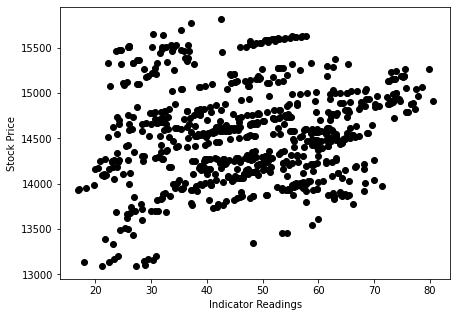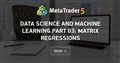# Discussion of article "Data Science and Machine Learning (Part 01): Linear Regression"

New article Data Science and Machine Learning (Part 01): Linear Regression has been published:

It's time for us as traders to train our systems and ourselves to make decisions based on what number says. Not on our eyes, and what our guts make us believe, this is where the world is heading so, let us move perpendicular to the direction of the wave.

Simple linear Regression has one dependent variable and one independent variable. Here we are trying to understand the relation between two variables for example, how does a stock price change with the change of a simple moving average

Complicated Data

Suppose we have random scattered indicator values when drawn against the stock price (something that happens in real life)In this case, our Indicator/independent variable may not be the good predictor of our stock price/dependent variable

Author: Omega J Msigwa

Thank you very much.  I've been waiting for an article series on this subject!

Max Brown #:
Thank you very much.  I've been waiting for an article series on this subject!

Just an uneducated opinion... I don't need science to tell that SMA has a very strong correlation with the price, it's literally the average price! Seems like "cheating" a bit to get a nice straight line in your examples but I'm not sure if there is much value in it. One could make an argument that the correlation coef values of close to 1 should be eliminated as they are probably derived directly from the price and hence don't really predict direction, instead just repeat what we can already see with a bare eye on a chart without a single indicator.

I made a mistake in Multiple regression calculation, kindly do not consider the models on multiple regression session as I'm currently working on updating the content

best regards

My numerous studies show that there is no predictive potential in linear regression in today's market.
There is still some predictive potential in parabolic regression, but only after parabolic channels have been recognized.
But there will come a time when this potential runs out. :((

Omega J Msigwa #:

I made a mistake in Multiple regression calculation, kindly do not consider the models on multiple regression session as I'm currently working on updating the content

best regardsData Science and Machine Learning part 03: Matrix Regressions
• www.mql5.com
This time our models are being made by matrices, which allows flexibility while it allows us to make powerful models that can handle not only five independent variables but also many variables as long as we stay within the calculations limits of a computer, this article is going to be an interesting read, that's for sure.

Hi,

Can I ask why total_data is set to 744? Why 744?

Satoshi Asakura #:

Hi,

Can I ask why total_data is set to 744? Why 744?

744/24 hours == 31 days, actually there is no reason for it you can choose the dataset size whatever you like

Ask if we keep y_nasdag_predicted. where to make jupyter Notebook
can process
thank you
Files:

anan seti #:
Ask if we keep y_nasdag_predicted. where to make jupyter Notebook
can process
thank you

Can you explain? what do you mean

Reason: